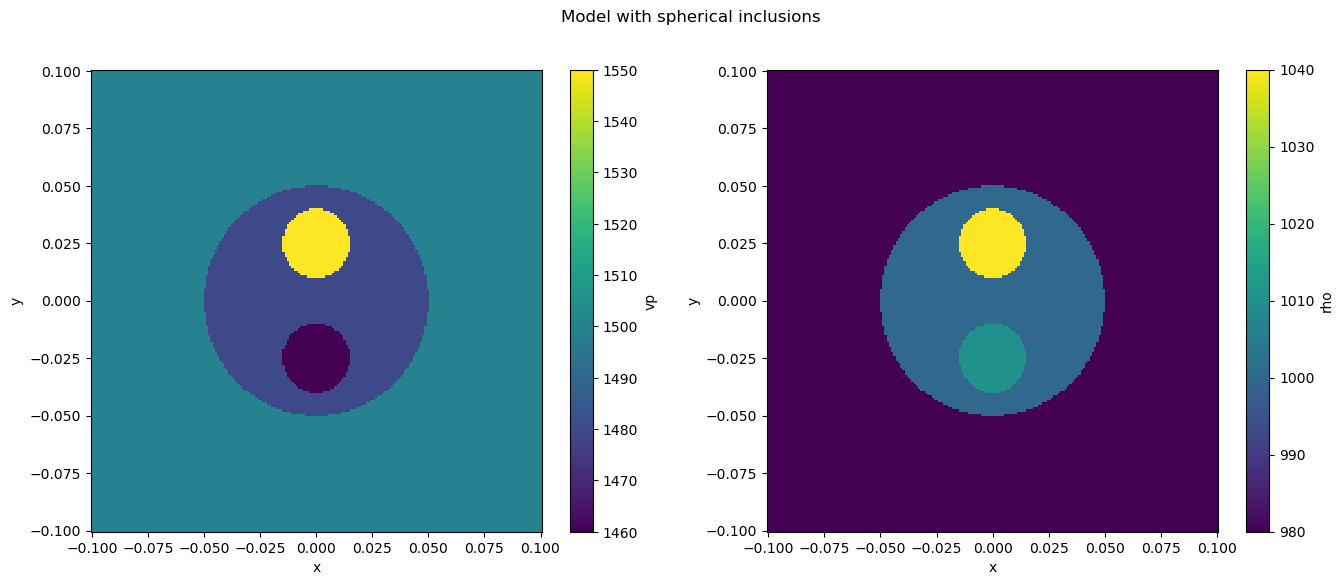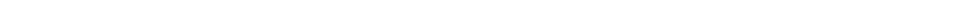Version:This tutorial is presented as Python code running inside a Jupyter Notebook, the recommended way to use Salvus. To run it yourself you can copy/type each individual cell or directly download the full notebook, including all required files.# Full-Waveform Inversion

## Part 1 - Setup and in-silico data

(Full)-Waveform Inversion (FWI) can reveal the properties of complex media that are otherwise not accessible to direct observation. This is based on measurements of mechanical waves - excited by either active or passive sources - that propagate through an object of interest and for which we record time series at certain receiver locations. Those data contain valuable information about the object's interior that we can use to create quantitative images of its material properties.
In this tutorial, we consider a fairly simple setup in 2D, which is inspired by typical apertures in ultrasound computed tomography (USCT) to illuminate human tissue.
Copy
%matplotlib inline

# This notebook will use this variable to determine which
# remote site to run on.
import os

SALVUS_FLOW_SITE_NAME = os.environ.get("SITE_NAME", "local")
import matplotlib.pyplot as plt
import numpy as np
import pathlib
import time
import xarray as xr

import salvus.namespace as sn

## Step 1: Generate the domain and ground truth

This is a purely synthetic study, so we have to generate the "measurements" ourselves using a model with a few inclusions. We use a simple box domain of 20 x 20 cm centered around the origin and insert an object with two spherical inclusions that mimics a breast phantom in a USCT acquisition. The model for density (RHO) and speed of sound (VP) is created from a structured grid.
def get_spherical_inclusion():
nx, ny = 200, 200
x = np.linspace(-0.1, +0.1, nx)
y = np.linspace(-0.1, +0.1, nx)
xx, yy = np.meshgrid(x, y, indexing="ij")

# Add 3 spherical inclusions
vp = 1500.0 * np.ones_like(xx)
rho = 980.0 * np.ones_like(xx)
mask = np.sqrt(xx**2 + yy**2) < 0.05

mask = np.sqrt(xx**2 + (yy - 0.025) ** 2) < 0.015

mask = np.sqrt(xx**2 + (yy + 0.025) ** 2) < 0.015

ds = xr.Dataset(
data_vars={
"vp": (["x", "y"], vp),
"rho": (["x", "y"], rho),
},
coords={"x": x, "y": y},
)

return ds
Let's take a look at the model.
true_model = get_spherical_inclusion()

# Plot the xarray dataset.
plt.figure(figsize=(16, 6))
plt.subplot(121)
true_model.vp.T.plot()
plt.subplot(122)
true_model.rho.T.plot()
plt.suptitle("Model with spherical inclusions")
plt.show()This is also the starting point for setting up a project for the inversion. We can directly create the project from the model defined above. We will call this model the true_model.
# Uncomment the following line to delete a
# potentially existing project for a fresh start
# !rm -rf project
vm = sn.model.volume.cartesian.GenericModel(name="true_model", data=true_model)
p = sn.Project.from_volume_model(
path="project",
volume_model=vm,
)## Step 2: Define the acquisition geometry

We assume a ring-shaped aperture with ultrasound transducers surrounding the breast phantom. To keep the computations cheap, we will use only 5 emitters and 100 receiving transducers which are the same for every emitter. The simple_config has a few built-in options to create lists of sources and receivers, which we want to make use of. By defining the two rings below - one for sources and one for the receivers, we can readily create an EventCollection, i.e., a number of experiments characterized by the locations of the emitting and the receiving transducers.
srcs = sn.simple_config.source.cartesian.collections.ScalarPoint2DRing(
x=0, y=0, radius=0.09, count=5, f=1.0
)

for _i, s in enumerate(srcs._sources):
x=0, y=0, radius=0.09, count=100, fields=["phi"]
)
recs = [
r
for r in all_recs._receivers
if np.sqrt(
(s.location - r.location) ** 2
+ (s.location - r.location) ** 2
)
> 0.03
]
p += sn.EventCollection.from_sources(
)
The transducers are identical except for their location, so we use the same simulation time of 1.5 ms (and later on also the same source time function) for each of them. Here, we also fix the time step to 400 ns. While this is not strictly necessary and could be automatically detected, of course, it will simplify the data comparison across different simulations.
wsc = sn.WaveformSimulationConfiguration(end_time_in_seconds=0.00015)
wsc.physics.wave_equation.time_step_in_seconds = 4.0e-7
Now, we put everything together to configure the simulations for the ground truth. To keep the costs low, we only consider a center frequency of 50 kHz and a mesh accurate up to 100 kHz.
ec = sn.EventConfiguration(
waveform_simulation_configuration=wsc,
wavelet=sn.simple_config.stf.Ricker(center_frequency=50000.0),
)
p += sn.SimulationConfiguration(
name="true_model_100kHz",
#
# Settings that influence the mesh.
elements_per_wavelength=2,
tensor_order=4,
max_frequency_in_hertz=100000.0,
#
model_configuration=sn.ModelConfiguration(
background_model=None, volume_models="true_model"
),
# Potentially event dependent settings.
event_configuration=ec,
)
Now it is time for the first simulation in our project. This is only to create data though. We haven't even started with the inversion yet...
p.simulations.launch(
simulation_configuration="true_model_100kHz",
events=p.events.get_all(),
site_name=SALVUS_FLOW_SITE_NAME,
ranks_per_job=1,
)
[2022-08-25 17:27:36,566] INFO: Creating mesh. Hang on.
[2022-08-25 17:27:37,472] INFO: Submitting job array with 5 jobs ...

5
p.simulations.query(block=True)True
p.simulations.get_mesh("true_model_100kHz")